Anúncio

# DFS.pptx

30 de Jan de 2023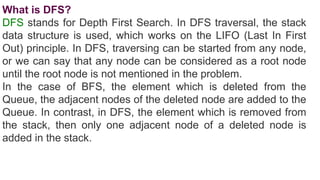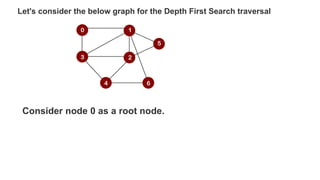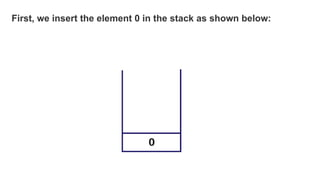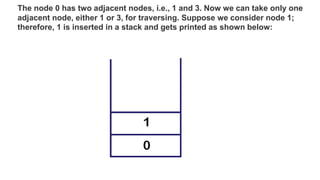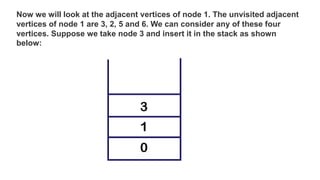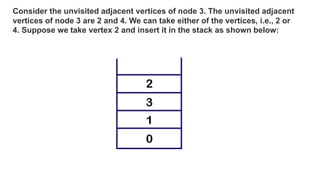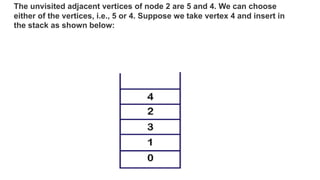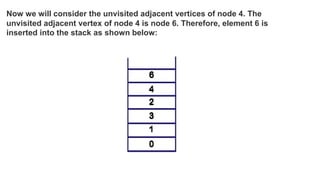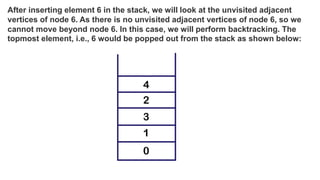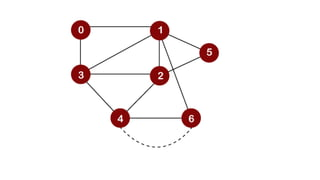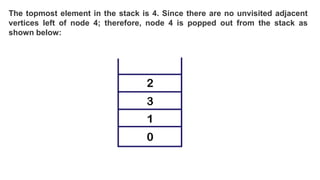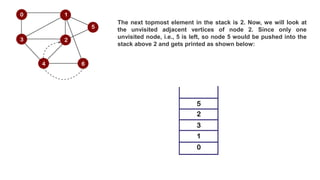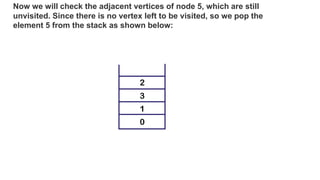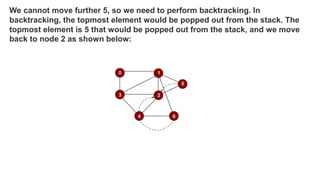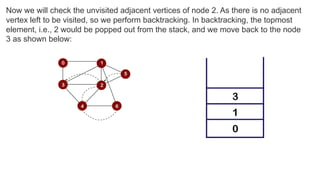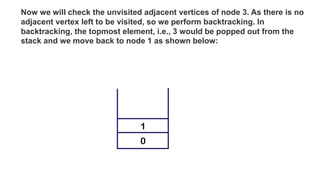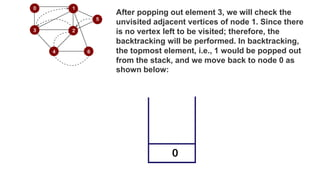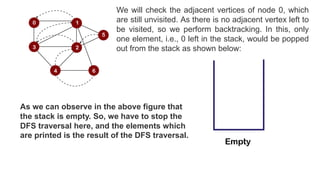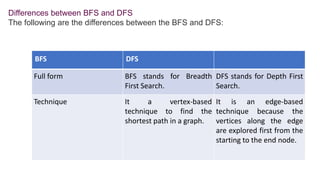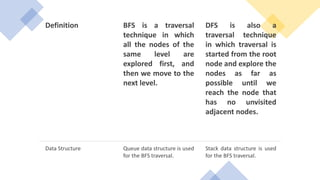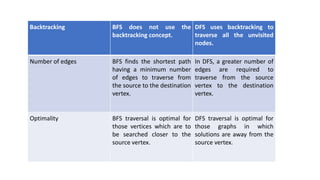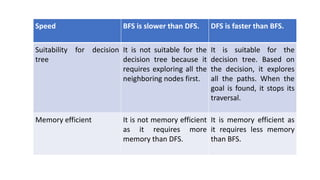1 de 22
Anúncio

### DFS.pptx

1. What is DFS? DFS stands for Depth First Search. In DFS traversal, the stack data structure is used, which works on the LIFO (Last In First Out) principle. In DFS, traversing can be started from any node, or we can say that any node can be considered as a root node until the root node is not mentioned in the problem. In the case of BFS, the element which is deleted from the Queue, the adjacent nodes of the deleted node are added to the Queue. In contrast, in DFS, the element which is removed from the stack, then only one adjacent node of a deleted node is added in the stack.
2. Let's consider the below graph for the Depth First Search traversal Consider node 0 as a root node.
3. First, we insert the element 0 in the stack as shown below:
4. The node 0 has two adjacent nodes, i.e., 1 and 3. Now we can take only one adjacent node, either 1 or 3, for traversing. Suppose we consider node 1; therefore, 1 is inserted in a stack and gets printed as shown below:
5. Now we will look at the adjacent vertices of node 1. The unvisited adjacent vertices of node 1 are 3, 2, 5 and 6. We can consider any of these four vertices. Suppose we take node 3 and insert it in the stack as shown below:
6. Consider the unvisited adjacent vertices of node 3. The unvisited adjacent vertices of node 3 are 2 and 4. We can take either of the vertices, i.e., 2 or 4. Suppose we take vertex 2 and insert it in the stack as shown below:
7. The unvisited adjacent vertices of node 2 are 5 and 4. We can choose either of the vertices, i.e., 5 or 4. Suppose we take vertex 4 and insert in the stack as shown below:
8. Now we will consider the unvisited adjacent vertices of node 4. The unvisited adjacent vertex of node 4 is node 6. Therefore, element 6 is inserted into the stack as shown below:
9. After inserting element 6 in the stack, we will look at the unvisited adjacent vertices of node 6. As there is no unvisited adjacent vertices of node 6, so we cannot move beyond node 6. In this case, we will perform backtracking. The topmost element, i.e., 6 would be popped out from the stack as shown below:
10. The topmost element in the stack is 4. Since there are no unvisited adjacent vertices left of node 4; therefore, node 4 is popped out from the stack as shown below:
11. The next topmost element in the stack is 2. Now, we will look at the unvisited adjacent vertices of node 2. Since only one unvisited node, i.e., 5 is left, so node 5 would be pushed into the stack above 2 and gets printed as shown below:
12. Now we will check the adjacent vertices of node 5, which are still unvisited. Since there is no vertex left to be visited, so we pop the element 5 from the stack as shown below:
13. We cannot move further 5, so we need to perform backtracking. In backtracking, the topmost element would be popped out from the stack. The topmost element is 5 that would be popped out from the stack, and we move back to node 2 as shown below:
14. Now we will check the unvisited adjacent vertices of node 2. As there is no adjacent vertex left to be visited, so we perform backtracking. In backtracking, the topmost element, i.e., 2 would be popped out from the stack, and we move back to the node 3 as shown below:
15. Now we will check the unvisited adjacent vertices of node 3. As there is no adjacent vertex left to be visited, so we perform backtracking. In backtracking, the topmost element, i.e., 3 would be popped out from the stack and we move back to node 1 as shown below:
16. After popping out element 3, we will check the unvisited adjacent vertices of node 1. Since there is no vertex left to be visited; therefore, the backtracking will be performed. In backtracking, the topmost element, i.e., 1 would be popped out from the stack, and we move back to node 0 as shown below:
17. We will check the adjacent vertices of node 0, which are still unvisited. As there is no adjacent vertex left to be visited, so we perform backtracking. In this, only one element, i.e., 0 left in the stack, would be popped out from the stack as shown below: As we can observe in the above figure that the stack is empty. So, we have to stop the DFS traversal here, and the elements which are printed is the result of the DFS traversal.
18. Differences between BFS and DFS The following are the differences between the BFS and DFS: BFS DFS Full form BFS stands for Breadth First Search. DFS stands for Depth First Search. Technique It a vertex-based technique to find the shortest path in a graph. It is an edge-based technique because the vertices along the edge are explored first from the starting to the end node.
19. Definition BFS is a traversal technique in which all the nodes of the same level are explored first, and then we move to the next level. DFS is also a traversal technique in which traversal is started from the root node and explore the nodes as far as possible until we reach the node that has no unvisited adjacent nodes. Data Structure Queue data structure is used for the BFS traversal. Stack data structure is used for the BFS traversal.
20. Backtracking BFS does not use the backtracking concept. DFS uses backtracking to traverse all the unvisited nodes. Number of edges BFS finds the shortest path having a minimum number of edges to traverse from the source to the destination vertex. In DFS, a greater number of edges are required to traverse from the source vertex to the destination vertex. Optimality BFS traversal is optimal for those vertices which are to be searched closer to the source vertex. DFS traversal is optimal for those graphs in which solutions are away from the source vertex.
21. Speed BFS is slower than DFS. DFS is faster than BFS. Suitability for decision tree It is not suitable for the decision tree because it requires exploring all the neighboring nodes first. It is suitable for the decision tree. Based on the decision, it explores all the paths. When the goal is found, it stops its traversal. Memory efficient It is not memory efficient as it requires more memory than DFS. It is memory efficient as it requires less memory than BFS.
Anúncio